# Foci and the Definitions of Ellipses and Hyperbolas

Lesson Transcript
Instructor: Laura Pennington

Laura received her Master's degree in Pure Mathematics from Michigan State University, and her Bachelor's degree in Mathematics from Grand Valley State University. She has 20 years of experience teaching collegiate mathematics at various institutions.

In this lesson, we'll look at the definition of an ellipse and a hyperbola. We'll use the foci of each of these to define them technically and formally, and we'll look at some examples to make the definitions more understandable. Updated: 03/21/2020

## Ellipses and Hyperbolas

Many of us are aware that the earth orbits the sun by traveling around it. However, what some people might not be aware of, is that the path the earth takes around the sun is the shape of a mathematical curve called an ellipse.

Wow! That's so cool! An ellipse is a mathematical shape that looks somewhat like a circle that has been squashed down a bit. Ellipses were first discovered by the ancient Greeks during their study of conic sections, where conic sections are curves that can be obtained by slicing a right cone at different angles. The ellipse is found by slicing a right cone as shown.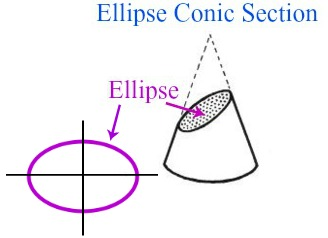Another conic section studied by the Greeks was the hyperbola. A hyperbola is a conic section that can be obtained by slicing a right cone as shown on screen now.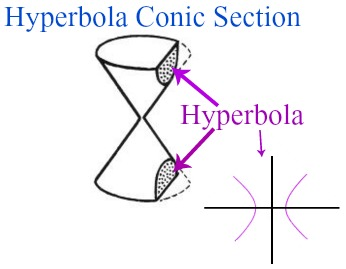When we slice the cone in this way, the cross section is a hyperbola and looks somewhat like two U's with their bottoms facing each other.

So far, this is pretty neat! Who would have though that slicing a cone could be so interesting! Let's take a look at a characteristic of both the ellipse and the hyperbola that will allow us to examine each curve more technically.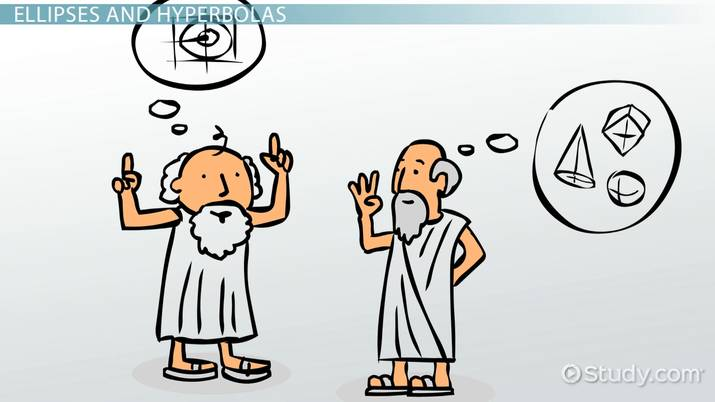An error occurred trying to load this video.

Try refreshing the page, or contact customer support.

Coming up next: Derive the Equation of an Ellipse from the Foci

### You're on a roll. Keep up the good work!

Replay
Your next lesson will play in 10 seconds
• 0:02 Ellipses and Hyperbolas
• 1:10 Foci of an Ellipse and…
• 2:46 Some Examples
• 5:02 Lesson Summary
Save Save

Want to watch this again later?

Timeline
Autoplay
Autoplay
Speed Speed

## Foci of an Ellipse and Hyperbola

Both an ellipse and a hyperbola have certain points called foci. Let's take a look at these points in each of the curves.

First, let's talk about the foci of an ellipse. The foci of an ellipse are two points, F and G, such that the distance from F to any point P, on the ellipse, to G is always the same.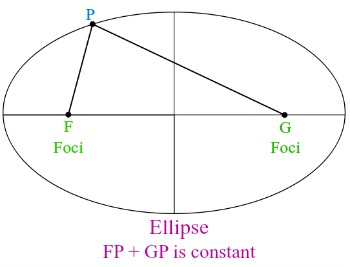This information allows us to give a more technical definition of an ellipse. That is, given two points, F and G, an ellipse is the set of points P, such that FP + PG is constant, and we call the points, F and G, the ellipse's foci.

As we said, it turns out that hyperbolas also have foci. To understand the foci of a hyperbola, it's best to define the hyperbola in terms of its foci. Doing this will also give us the technical definition of a hyperbola, so let's give it a go!

For two given points, F and G called the foci, a hyperbola is the set of points, P, such that the difference between the distances, FP and GP, is constant. That is, if F and G are the foci of a hyperbola, then for any point, P, on the hyperbola, the absolute value of FP - GP is constant.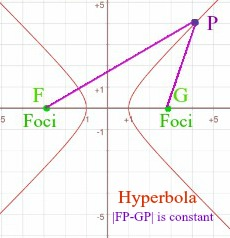Hmmm…that's a lot of information. I don't know about you, but I prefer to just think of an ellipse as a squashed circle and a hyperbola as two U's with their bottoms facing each other. Nonetheless, knowing what the foci is for each of these curves, along with their technical definitions, is very important when studying these concepts.

## Some Examples

Let's take a look at a couple of examples to further our understanding of ellipses and hyperbolas.

First, consider the ellipse shown with foci (-4, 0) and (4, 0). The image shows this ellipse with two points on the ellipse labeled.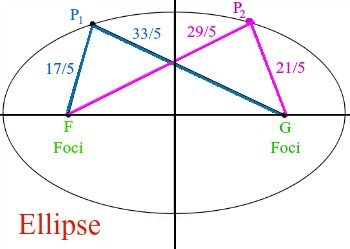Notice that the distances from each of the foci to the points on the ellipse are as follows:

FP1 = 17 / 5

To unlock this lesson you must be a Study.com Member.

### Register to view this lesson

Are you a student or a teacher?